### Home > MC1 > Chapter 6 > Lesson 6.1.4 > Problem6-39

6-39.
1. . Answer each of the questions below. Homework Help ✎

1. How many fourths are in 4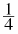?
Use your answer to rewrite 4in the form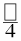2. How many thirds are in 3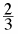?
Use your answer to rewrite 3in the form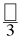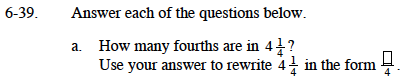If there are 4 fourths in 1, can you use multiplication to find how many fourths are in 4?

Using your answer to Step 1, add the number of fourths in 4 to the one-fourth in part (a).

$\text{16\ fourths + 1\ fourth = \frac{?}{4}}$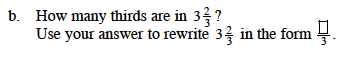$\text{There are 11 thirds in }3\frac{2}{3},\text{ so }3\frac{2}{3}=\frac{11}{3}$